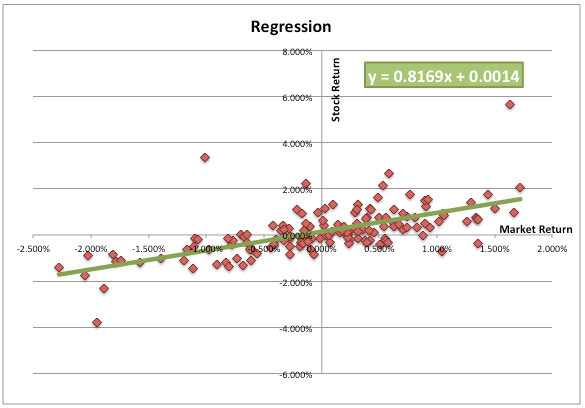# Calculating Beta Using Market Model Regression (Slope)

While calculating the cost of equity, it is important for an analyst to calculate the beta of the company’s stock.

Beta of a publicly traded company can be calculated using the Market Model Regression (Slope).

In this method, we regress the company’s stock returns (ri) against the market’s returns (rm). The beta (β) is represented by the slope of the regression line.

$r_{i}=\alpha +\beta r_{m}$

Where,

ri is the stock’s return

α represents the intercept

β is the stock’s beta

rm is the market returnsSome of the issues while calculating beta are discussed below:

1. Estimation period:  Beta is usually estimated using the historical data for 2-9 years. The choice of estimation period affects the calculation of Beta. If the estimation period is short, it does reflect the current dynamics of the company. For example, for a company that has recently undergone structural changes, it’s better to use short estimation period for calculating beta. However, for a company that has a stable operating history, a long estimation period will be suitable.
2. Return interval: An analyst may take daily, weekly, or monthly returns of the stock and market while performing regression. Generally a shorter observation period such as daily returns leads to lower standard error.
3. Choice of the market index: The analyst also needs to carefully choose a market index to regress the returns against.
4. Smoothing techniques: Betas also have a tendency to revert to 1. Analysts may have to use some smoothing techniques to adjust the beta.
5. Small-cap stocks: The small cap stocks tend to be more risk with a higher return potential. Therefore, analysts may want to adjust the beta pf small cap stock upwards.

Using the above methods, we can easily estimate the beta of a publicly traded company, as all the information such as historical returns is publicly available. The problem arises when an analyst is required to estimate the beta of a non-public company or a project, for which there is no historical stock return data. The analyst may have to use a proxy of the beta by using some combination of the information available about the company/project and a comparable public limited company. A commonly used method is the pure-play method, in which an analyst observes the beta of a comparable public company, and adjusts it for the difference in financial leverage.